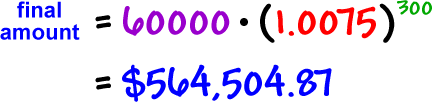What if we made the same investment, but compounded it quarterly?

initial amount = 60,000

At the end of each period (every 3 months), we'll be earning 2.25%...  (That's 9% divided by 4  >>>  .09 / 4 = .0225)
So, each
\$1.00 will turn into \$1.0225

growth factor = \$1.0225

number of periods = 100See how we made more money?  Will we make even more if we compounded it monthly?

initial amount = 60,000

At the end of each period (every month), we'll be earning 0.75%
(That's 9% divided by 12  >>>  .09 / 12)
So, each
\$1.00 will turn into \$1.0075...

growth factor = \$1.0075

number of periods = 300So, the more times we compound, the more money we make!!  Cool.